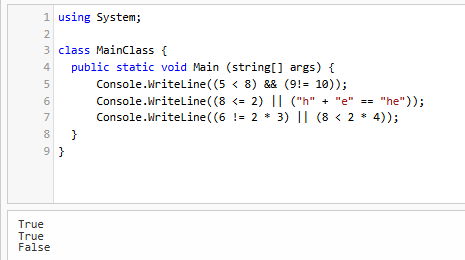# Boolean Operators

## Booleans Operators

You can also connect boolean expressions together using the && (AND) and the || (OR) operator. For example, suppose I ask: “Are you a human, and is Nuvi a robot?” The word “and” connects the two true-false questions together. In this case, since it is true that you are a human, and it is also true that Nuvi is a robot, then the overall result is true.

Here’s a chart that describes what happens when we connect booleans together:

ExpressionResultExpressionResult
true && truetruetrue || truetrue
true && falsefalsetrue || falsetrue
false && truefalsefalse || truetrue
false && falsefalsefalse || falsefalse

To summarize, && requires both Boolean expressions to be true, while || only requires one of the two Boolean expressions to be true. Here are some more examples:

• (5 < 8) && (9 != 10) produces true since both 5 is less than 8 and 9 is not equal to 10.
• (8 <= 2) || ("h" + "e" == "he") produces true since "h" + "e" results in "he", even though 8 is not less than or equal to 2.
• (6 != 2 * 3) || (8 < 2 * 4) produces false since both 6 not equal to 2 * 3, and 8 not being less than 2 * 4, produce false.